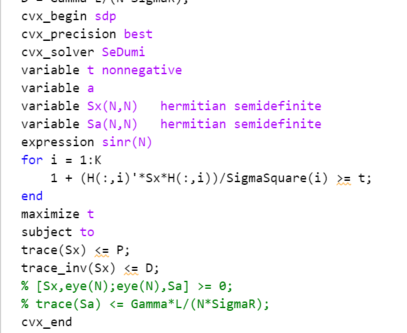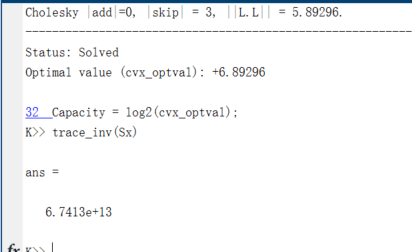# A problem when using trace_inv function in a constraint with CVX

Hello, every! I have come across a problem when using CVX to solve a multicast capacity calculation problem.P and D are both constant values. As we all know, the trace(.^-1) is a strict convex function and in this case, I need to make a constraint on the trace(Sx^-1) thus I use the trace_inv function in my constraints. This code actually works and return the cvx_status as ‘Solved’. However, when I check the obtained solution Sx, I found that it is not full-rank and thus the trace(Sx^-1) is infinite. Obviously the constraint using trace_inv function is not satisfied. What is the reason for this situation and how can I rewrite this trace_inv constraint? I have tried schur component for this formula and get similar problem. But if the trace_inv is used in objective function, it seems get correct outputs.Here is the cvx_status and a very large trace_inv.

Can you provide your data (e.g.,P,D,H,etc) and your code in a markdown format, so we can reproduce your problem?

N = 8;
K = 4;
H = sqrt(1/2)(randn(AntennaNum,UserNum) + randn(AntennaNum,UserNum)1i);
SigmaR = 1;
SigmaK = ones(UserNum, 1);
P = 1;
L = 256;
CRBThreshold = 1;
[Capacity,Sx] = CalculateCapacity(H, L, SigmaK, SigmaR, CRBThreshold, K, N, P)
function [Capacity, CovarMatrix] = CalculateCapacity(ChannelMatrix, TimeBlockLength, NoisePowerCU, NoisePowerTarget, CRBThreshold, UserNum, …
AntennaNum, Power)
%CalculateCapacity 计算可达容量上界
% 输出相关矩阵与容量
H = ChannelMatrix;
L = TimeBlockLength;
K = UserNum;
N = AntennaNum;
SigmaSquare = NoisePowerCU;
SigmaR = NoisePowerTarget;
Gamma = CRBThreshold;
P = Power;
D = Gamma
L/(N
SigmaR);
cvx_begin sdp
cvx_precision best
cvx_solver SeDumi
variable t nonnegative
variable Sx(N,N) hermitian semidefinite
variable Sa(N,N) hermitian semidefinite
expression sinr(N)
maximize t
subject to
for i = 1:K
1 + (H(:,i)'SxH(:,i))/SigmaSquare(i) >= t;
end
trace(Sx) <= P;
trace_inv(Sx) <= D;
% [Sx,eye(N);eye(N),Sa] >= 0;
% trace(Sa) <= GammaL/(NSigmaR);
cvx_end
Capacity = log2(cvx_optval);
CovarMatrix = Sx;
end

what is the value of AntennaNum,UserNum? pls provide their values so we can run your code.

It means antenna number and communication user number

``````N = 8;
K = 4;
H = sqrt(1/2)*(randn(AntennaNum,UserNum) + randn(AntennaNum,UserNum)*1i);
SigmaR = 1;
SigmaK = ones(UserNum, 1);
P = 1;
L = 256;
CRBThreshold = 1;
[Capacity,Sx] = CalculateCapacity(H, L, SigmaK, SigmaR, CRBThreshold, K, N, P)
function [Capacity, CovarMatrix] = CalculateCapacity(ChannelMatrix, TimeBlockLength, NoisePowerCU, NoisePowerTarget, CRBThreshold, UserNum, ...
AntennaNum, Power)
%CalculateCapacity 计算可达容量上界
%   输出相关矩阵与容量
H = ChannelMatrix;
L = TimeBlockLength;
K = UserNum;
N = AntennaNum;
SigmaSquare = NoisePowerCU;
SigmaR = NoisePowerTarget;
Gamma = CRBThreshold;
P = Power;
D = Gamma*L/(N*SigmaR);
cvx_begin sdp
cvx_precision best
cvx_solver SeDumi
variable t nonnegative
variable Sx(N,N)   hermitian semidefinite
variable Sa(N,N)   hermitian semidefinite
expression sinr(N)
maximize t
subject to
for i = 1:K
1 + (H(:,i)'*Sx*H(:,i))/SigmaSquare(i) >= t;
end
trace(Sx) <= P;
trace_inv(Sx) <= D;
% [Sx,eye(N);eye(N),Sa] >= 0;
% trace(Sa) <= Gamma*L/(N*SigmaR);
cvx_end
Capacity = log2(cvx_optval);
CovarMatrix = Sx;
end``````

This is not a runnable code. Obviously many elements are missing. and you use random data which is not provided.

Sorry I actually made a silly mistake on the variable definition!! Now the code works. Anyway, thanks for your reply